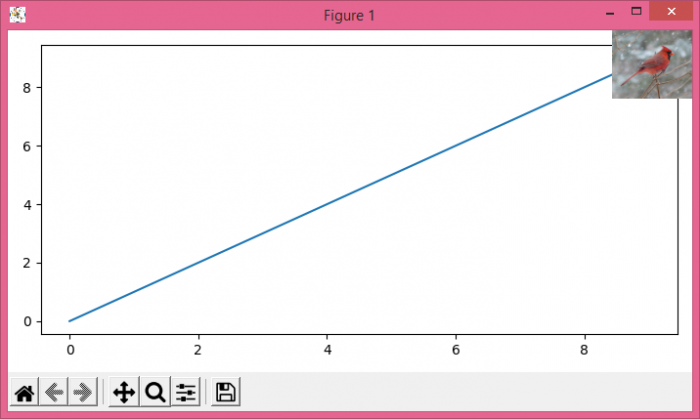# How to insert a small image on the corner of a plot with Matplotlib?

To insert a small image on the corner of a plot with matplotlib, we can take the following steps−

• Read an image from a file into an array using imread() method.
• Using subplots() method, create a figure and add a set of subplots.
• Plot a line on the current axis.
• Create newax (new axis) to show the image array (Step 1).
• Turn off the newly created axis, created for an image insert.
• To display the figure, use show() method.

## Example

import matplotlib.pyplot as plt
plt.rcParams["figure.figsize"] = [7.00, 3.50]
plt.rcParams["figure.autolayout"] = True
im = plt.imread('bird.jpg') # insert local path of the image.
fig, ax = plt.subplots()
ax.plot(range(10))
plt.show()